# What is Analog to Analog Conversion (Modulation)?

Computer NetworkNetworkOperating System

It is the description of analog data by an analog signal. Modulation of analog signals means converting analog signals to an analog signal. It is required because the sender’s signal is of low pass and can be of the same range. For example, each radio station has a low pass signal, which may be of the same range. Different stations signal to avoid intermixing; each low pass signal must be shifted to a diverse range on the frequency band.

The diagram shows the relationship between the analog information, the analog-to-analog conversion hardware, and the resultant analog signal.

Analog-to-Analog modulation can be achieved in three ways as under −

## Amplitude Modulation

In AM transmission, the carrier signal modulates to vary with the modulation signal’s amplitude.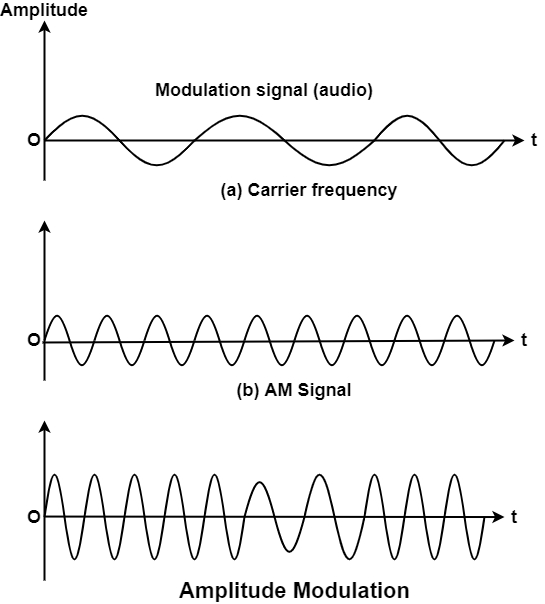It can be noted that in amplitude modulation, the frequency and phase of the carrier remain constant. The amplitude modifies to follow variations in the data or message signal. The figure shows the concept of the amplitude modulation process. It can be observed that here the modulation signal turn into an envelope to the carrier.

## Bandwidth of Amplitude Modulation (AM) Signal

The bandwidth of an AM signal is the same as twice the bandwidth of the modulating signal and wrap a range centred on the carrier frequency, as shown in the diagram.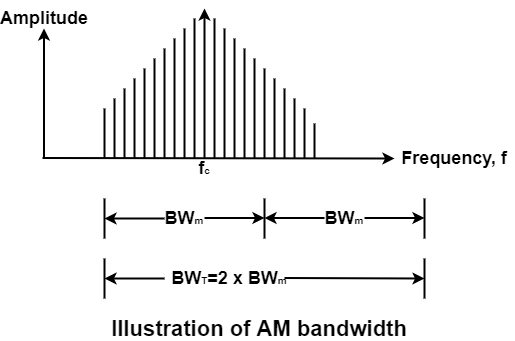The line portion of the graph is known as the frequency spectrum of the signal. Usually, the bandwidth of an audio signal (i.e., speed and music) is 5 KHz. Hence, it can conclude that an AM radio station needed a minimum bandwidth of 10 KHz. The Federal communication commission (FCC) enables 10 KHz for each AM station.

AM stations enable carrier frequencies anywhere between 530 KHz and 1700 KHz (i.e., 1.7 MHz). But each station carrier frequency should be separated from those on either side of it by at last 10 KHz (one AM bandwidth) to prevent interference. As one KHz, the next station’s carrier frequency cannot be reduced to 1110 KHz, as shown in the diagram.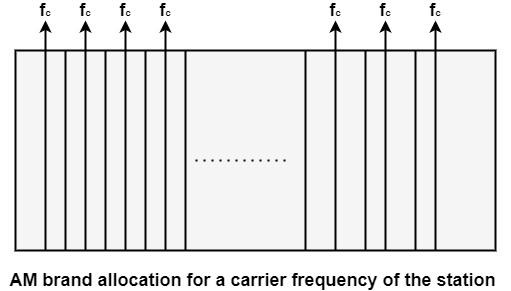Thus, the total bandwidth needed for AM can be resolved from the bandwidth of the audio signal −

$$B_{T}=2\times\:B_{A}$$

## Frequency Modulation

In FM transmission, the carrier signal frequency is modulated (i.e., varied) according to the voltage level variations (i.e., amplitude) of the modulation signal.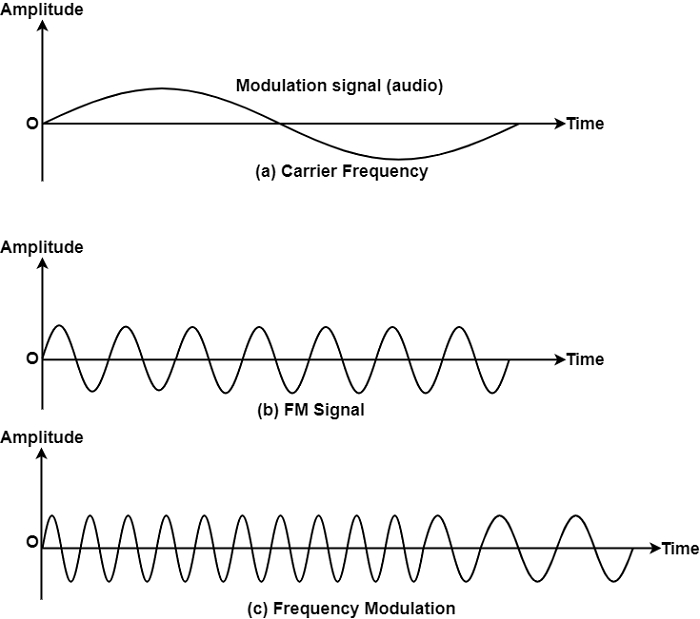The carrier signal’s peak amplitude and phase remain constant, but as the signal amplitude changes, the carrier’s frequency changes correspondingly. The figure displays the modulation signal’s relationships, the carrier signal, and the resultant FM signal.

## FM Bandwidth

The bandwidth of an FM signal is similar to 10 times the bandwidth of the modulating signal and, like AM bandwidths, covers a range centred on the carrier frequency. The figure displays both the bandwidth and the shaded area, the frequency spectrum of an FM signal. The bandwidth of an audio signal (speech and music) broadcast in stereo is around 15 KHz. Each FM radio station, therefore, requires a minimum of 150 KHz.

The FCC enable 200 KHz (0.2 MHz) to provide room for guard bands for each station. FM stations are granted carrier frequencies anywhere between 88 and 108 MHz. Stations should be divided by a minimum of 200 KHz to hold their bandwidth from overlapping.

The other remains unused to avoid any feasibility of two stations intrusive with each other. Consider 88 to 108 MHz as a range, and there is 100 potential FM bandwidth in an area where 50 can work at any time.

The total bandwidth needed for FM can be determined from the bandwidth of the audio signal.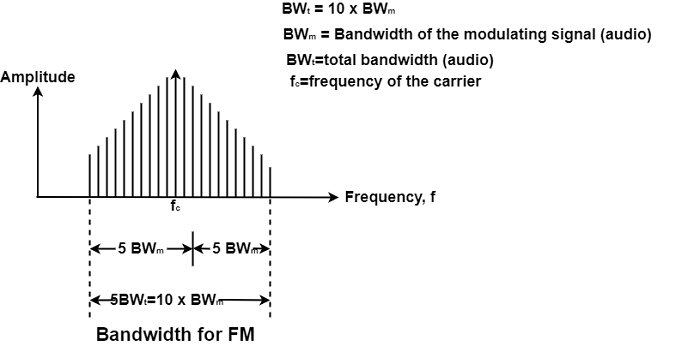The bandwidth of a stereo audio signal is generally 15 KHz. Hence, an FM station requires at least a bandwidth of 150 KHz. The FCC needed the minimum bandwidth to be a minimum of 200 KHz (0.2 MHz)

$$f_{c}=carrier\:frequency\:of\:the\:station$$ fm_bandwidth.jpg

## Phase Modulation (PM)

Because of simpler hardware requirements, phase modulation (PM) is used in some system as different to frequency modulation. In PM transmission, the carrier signal phase is modulated to follow the modulating signal’s changing voltage level (amplitude).

The carrier signal’s peak amplitude and frequency remain consistent, but as the amplitude of the data signal changes, the process of the carrier changes correspondingly. The analysis and the outcomes (modulated signal) are similar to those of frequency modulation.